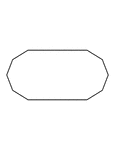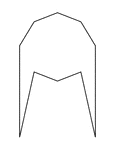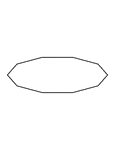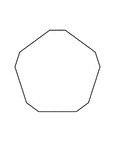### Irregular Convex Decagon

Illustration of an irregular convex decagon. This polygon has some symmetry.### Irregular Convex Decagon

Illustration of an irregular convex decagon. This polygon has some symmetry.### Irregular Convex Decagon

Illustration of an irregular convex decagon. This polygon has some symmetry.### Irregular Convex Decagon

Illustration of an irregular convex decagon. This polygon has some symmetry.### Irregular Convex Decagon

Illustration of an irregular convex decagon. This polygon has some symmetry.